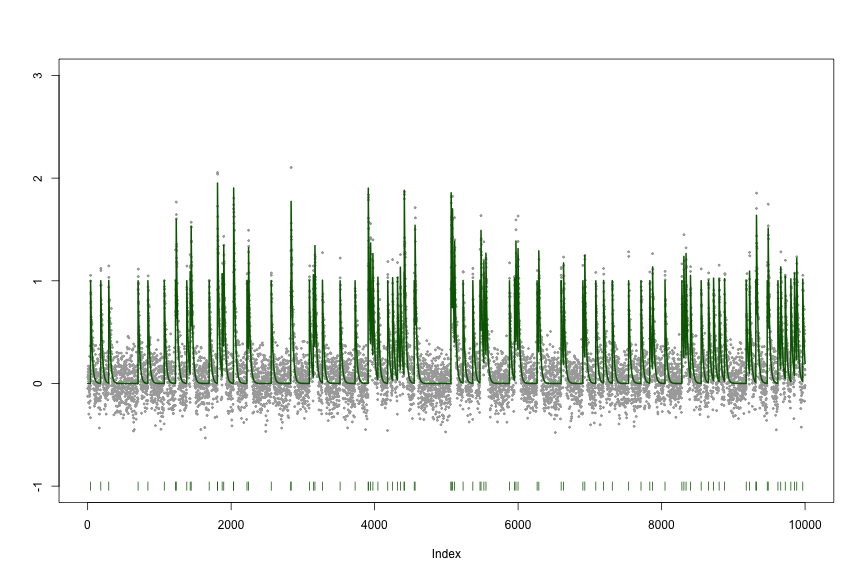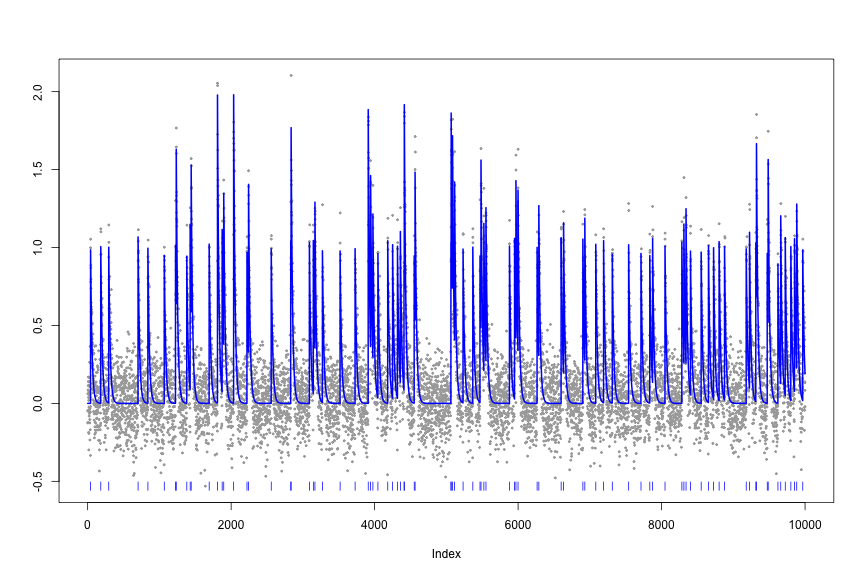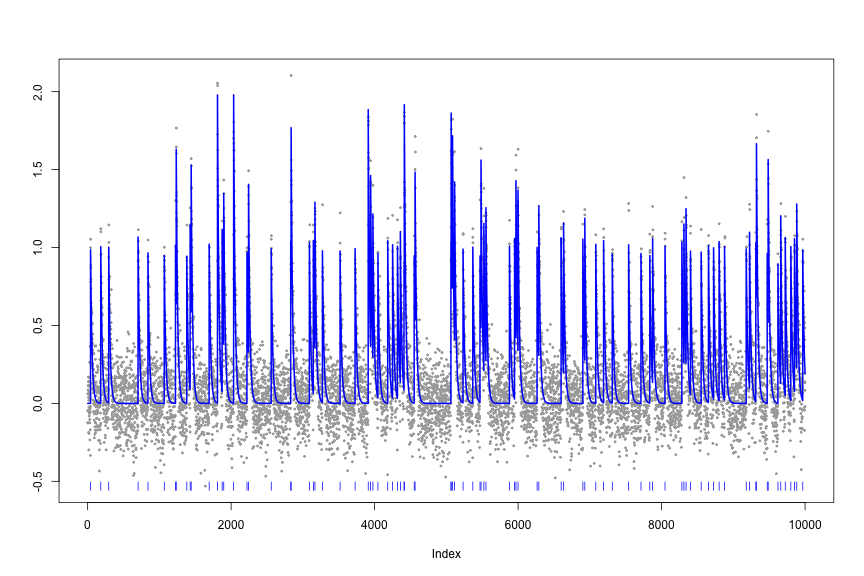## R tutorial

In this tutorial, we demonstrate how the FastLZeroSpikeInference package can be used to deconvolve calcium imaging data from a single neuron. All demos require the FastLZeroSpikeInference package; installation instructions are provided here.

To illustrate the software, we generate a synthetic dataset according to

where $\epsilon_t \sim \mathrm{N}(0, \sigma^2)$ and $c_1 = 1$. The function simulate_ar1 generates data from this model.The estimate_spikes function estimates spikes based on the calcium trace, exponential decay parameter $\gamma$, and a tuning parameter $\lambda$. This function solves

if the constraint parameter is set to false (default), and

if the constraint parameter is set to true. Here’s an example of the function applied to the previously generated data.

By default, and to save computation time, the calcium concentration is not automatically estimated. However, the estimate_calcium function can be used to estimate the calcium concentration based on a prior fit.Alternatively, both spikes and calcium concentrations can be computed with the estimate_spikes function with estimate_calcium parameter set to true.To solve the constrained problem

we set the constraint parameter to true in the estimate_spikes function. Consider the following example.